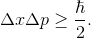# GRE Subject Test: Physics : Quantum Mechanics

## Example Questions

### Example Question #1 : Quantum Mechanics And Atomic Physics

Which of the following wavelengths could be used to measure the position of an electron with the greatest accuracy?

Infared

Gamma-rays

Red light

X-ray

Gamma-rays

Explanation:

The Heisenberg Uncertainty principle states thatTherefore, the smallest wavelength will have the lowest uncertainty in the position. Thus, we must pick the regime of options given which has the lowest wavelength. That is gamma-ray.

### Example Question #2 : Quantum Mechanics And Atomic Physics

What wavelength will result in the most accurate measurement of the momentum of an electron?

X-ray

Gamma-ray

Red light

Infrared

Explanation:

The Heisenberg Uncertainty Principle states that:Since the uncertainty in the position is inversely proportional to the uncertainty in the momentum, we need to pick the longest wavelength. Of the options listed, radio has the longest wavelength.

### Example Question #1 : Square Wells/Particle In A Box

If a ground state particle is in a one-dimension square well, where is the probability of finding the particle equal to zero?

At the boundary.

In the middle.the way in the box.the way in the box.

None of these